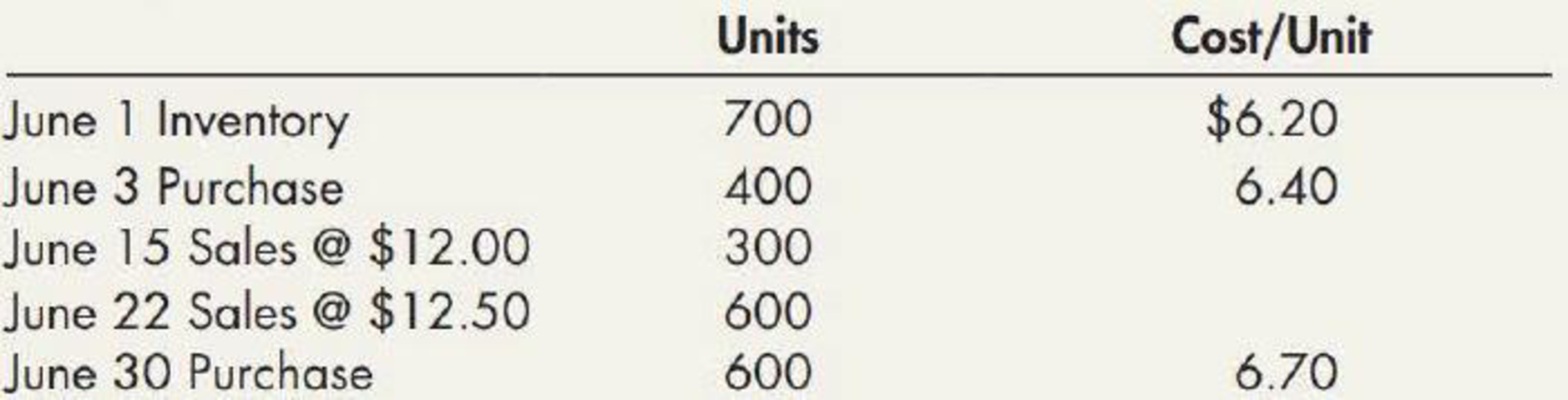Chapter 7, Problem 14E### Intermediate Accounting: Reporting...

3rd Edition
James M. Wahlen + 2 others
ISBN: 9781337788281

#### Solutions

Chapter
Section### Intermediate Accounting: Reporting...

3rd Edition
James M. Wahlen + 2 others
ISBN: 9781337788281
Textbook Problem
90 views

# LIFO, Perpetual and Periodic Riedel Company’s inventory records showed the following transactions for the fiscal period ended June 30:Required: 1. Compute the ending inventory and the cost of goods sold under the LIFO cost flow assumption assuming both a perpetual and a periodic inventory system. 2. Next Level Explain the difference in the final inventory valuations.

1)

To determine

Determine the ending inventory and the cost of goods sold under LIFO method assuming both perpetual and a periodic inventory system.

Explanation

LIFO: In Last-in-First-Out method, items purchased recently are sold first. So, the value of the ending inventory consist the initial cost for the remaining unsold items.

Periodic inventory system: The method or system of recording the transactions related to inventory occasionally or periodically is referred as periodic inventory system.

Determine the ending inventory using LIFO method using periodic inventory system:

 Particulars Units Price per unit Amount Beginning inventory on June 01 700 $6.20$4,340 Add: Inventory purchased on June 03 100 $6.40$640 Ending inventory 800 $4,980 Table (1) Determine the cost of goods sold using LIFO method using periodic inventory system: Cost of goods sold}=[Beginning inventory]+[Net purchases][Ending inventory]=[700×$6.40]+[(700×$6.40)+(600×$6

2)

To determine

Elaborate the difference in the final inventory valuation.

### Still sussing out bartleby?

Check out a sample textbook solution.

See a sample solution

#### The Solution to Your Study Problems

Bartleby provides explanations to thousands of textbook problems written by our experts, many with advanced degrees!

Get Started